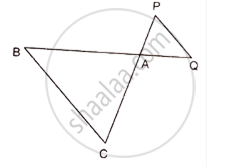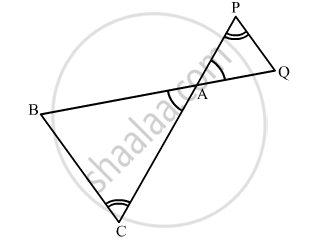Share

# In figure, ∆ACB ~ ∆APQ. If BC = 8 cm, PQ = 4 cm, BA = 6.5 cm, AP = 2.8 cm, find CA and AQ. - Mathematics

Course

#### Questions

In figure, ∆ACB ~ ∆APQ. If BC = 8 cm, PQ = 4 cm, BA = 6.5 cm, AP = 2.8 cm, find CA and AQ.

In the following figure, ΔACB ~ ΔAPQ. If BC = 8 cm, PQ = 4 cm, BA = 6.5 cm and AP = 2.8 cm, find CA and AQ.#### Solution

We have, ∆ACB ~ ∆APQ\Rightarrow \frac{AC}{AP}=\frac{CB}{PQ}=\frac{AB}{AQ}

\Rightarrow \frac{AC}{AP}=\frac{CB}{PQ}\text{ and }\frac{CB}{PQ}=\frac{AB}{AQ}

\Rightarrow \frac{AC}{2.8}=\frac{8}{4}\text{ and }\frac{8}{4}=\frac{6.5}{AQ}

\Rightarrow \frac{AC}{2.8}=2\text{ and }\frac{6.5}{AQ}=2

AC = (2 × 2.8) cm = 5.6 cm and AQ=\frac{6.5}{2}cm=3.25cm

Is there an error in this question or solution?

#### APPEARS IN

RD Sharma Solution for Class 10 Maths (2018 (Latest))
Chapter 7: Triangles
Ex. 7.5 | Q: 1 | Page no. 73
RD Sharma Solution for Class 10 Maths (2018 (Latest))
Chapter 7: Triangles
Ex. 7.5 | Q: 1 | Page no. 73